# TSA Principal Component Analysis VI

LabVIEW 2014 Advanced Signal Processing Toolkit Help

Edition Date: June 2014

Part Number: 372656C-01

»View Product InfoDownload Help (Windows Only)

Owning Palette: Statistical Analysis VIs

Performs principal component analysis (PCA) on a multivariate (vector) time series. Wire data to the Xt input to determine the polymorphic instance to use or manually select the instance.

Use the pull-down menu to select an instance of this VI.

 Select an instance TSA Principal Component Analysis (Waveform)TSA Principal Component Analysis (Array)

## TSA Principal Component Analysis (Waveform)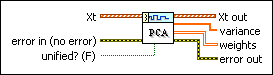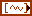Xt specifies the multivariate (vector) time series.error in describes error conditions that occur before this node runs. This input provides standard error in functionality.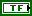unified? specifies to remove the mean and then unify Xt before performing PCA. The default is FALSE.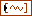Xt out returns the computed principal component scores.variance returns the variance values of the calculated principal component scores.weights returns the weight matrix that this VI used when computing principal component scores from multivariate time series.error out contains error information. This output provides standard error out functionality.

## TSA Principal Component Analysis (Array)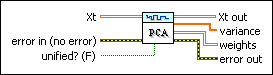Xt specifies the multivariate (vector) time series. Each column of the 2D array represents a vector at certain time.error in describes error conditions that occur before this node runs. This input provides standard error in functionality.unified? specifies to remove the mean and then unify Xt before performing PCA. The default is FALSE.Xt out returns the computed principal component scores. Each column of the 2D array represents a series of the computed principal component scores.variance returns the variance values of the calculated principal component scores.weights returns the weight matrix that this VI used when computing principal component scores from multivariate time series.error out contains error information. This output provides standard error out functionality.

## TSA Principal Component Analysis Details

Principal component analysis (PCA) computes a new set of uncorrelated multivariate (vector) time series by a transform of coordinate rotation from original correlated multivariate time series.

This VI performs PCA according to the following steps:

1. Calculates the covariance/correlation coefficient matrix

This VI calculates the covariance matrix according to the following equation:where w = N–1, N is the number of time series samples. The dimension of the covariance matrix is m×m.

This VI calculates the correlation coefficient matrix according to the following equation: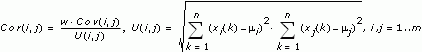If unified? is TRUE, this VI calculates the correlation coefficients matrix first and then performs PCA. If unified? is FALSE, this VI calculates the covariance matrix first, and then performs PCA.

2. Calculates the eigenvectors and eigenvalues of the matrix

This VI obtains the m eigenvalues li and eigenvectors vi from either covariance matrix or correlation matrix. li and vi satisfy the following equation:

AVi = liVi, i=1, …, m

where A is an m×m covariance matrix or correlation matrix.

3. Generates the new time series

This VI then computes a new set of uncorrelated multivariate (vector) time series according to the following equation:

Xtnew=VTXt

where Xtnew is the new uncorrelated multivariate (vector) time series, and Xt is the original correlated multivariate (vector) time series. Both of them are m×n matrices whose row vectors represent a single channel time series. V is eigenvectors matrix [v1, v2,…, vm].

V also is the weight matrix. Each column of V is one principle component. Xtnew also is the principal component scores. Each row of Xtnew is the scores for one principal component. Each li is variance of the scores for one principal component.

## Examples

Refer to the following VIs for examples of using the TSA Principal Component Analysis VI:

• Principal Component Analysis VI: labview\examples\Time Series Analysis\TSAGettingStarted
• Image Compression with PCA VI: labview\examples\Time Series Analysis\TSAApplications